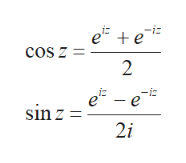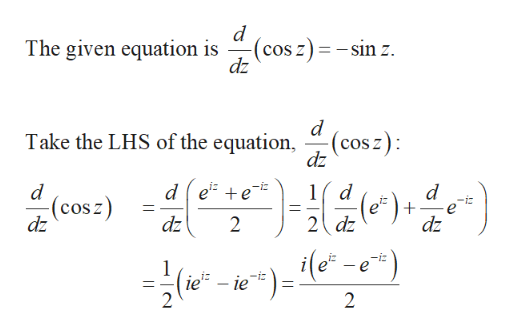a+ b70 Complex NumbersChapter 212. HYPERBOLIC FUNCTIONSLet us look at sinz and cos z for pure imaginary z, that is, z=iy:e-ee-esin iy=2i(12.1)e ee eCOs iy2The real functions on the right have special names because these particular combi-nations of exponentials arise frequently in problems. They are called the hyperbolicsine (abbreviated sinh) and the hyperbolic cosine (abbreviated cosh). Their defini-tions for all z aree-esinhz(12.2)Cosh z = +eThe other hyperbolic functions are named and defined in a similar way to parallelthe trigonometric functions:sinhzcoshcoth tanh ztanh(12.3)1csehz=sinhz'sech:cosh(See Problem 38 for the reason behind the term "hyperbolic" functions.)We can write (12.1) assin iy i sinh y,cos iy cosh y.(12.4)Then we see that the hyperbolic functions of y are (except for one i factor) thetrigonometric functions of iy. From (12.2) we can show that (12.4) holds with yreplaced by . Because of this relation between hyperbolic and trigonometric functions, the formulas for hyperbolic functions look very much like the correspondingtrigonometric identities and calculus formulas. They are not identical, however.Example. You can prove the following formulas (see Problems 9, 10, 11 and 38).cOsh2-sinh+ cos? z = 1),1(compare sin2dcosh zsinh z(compare cos z=-sinz).dzPROBLEMS, SECTION 12Verify each of the following by using equations (11.4), (12.2), and (12.3).sin zsin(z+iy)=sinz cosh y+ i cosr sinh y1.Section 1271Hynerholic Functions (comparecos z = - sin z).COsh zsinh zCOsPROBLEMS, SECTION 12Verify each of the following by using equations (11.4), (12.2), and (12.3)1.sin zsin(z+iy) = sinz cosh y + i cosr sinh yHyperbolic Functions 71Section 12cos zcosr cosh y - i sinz sinh ysinhz=sinhz cos y+i cosh z sin y2.3.cosh zcoshr cos yi sinhz sin y4sin 2z=2 sin z cos5.COs 2\$+/sinh 2z 2 sinh z cosh zcos 2zcos :- sin2 z7.6.cosh 2zcosh2 z + sinh2 z9.COs z=sin zdz8.d10. csh z =:coshz-sinh2 : = 1sinh z11.cos+sin= 1-; sin 2Cos 3z= 4 cas"-3cos12.13.sinhizi sin zsin iz = i sinh14.15.17. tanh iz = i tan 2taniz = i tanh z16.tanz+itanhytan:= tan(z + iy) = 1 r i tan s tanh y18.tanhz+i tanytanhz=1+i tanh z tan y19.Show that ez = (coshz sinh z )" = cosh nz + sinh nz. Use this and a similarequation for e-n* to find formulas for cosh 3z and sinh 3z in terms of sinh z andcosh z20.21.Use a computer to plot graphs of sinh r, cosh z, and tanh z22.Using (12.2) and (8.1), find, in summation form, the power series for sinhz andcosh r. Check the first few terms of your series by computer.Find the real part, the imaginary part, and the absolute value of23. cosh(iz)24. cos(iz)25. sin(-iy)28. tanh(1-in)26. cosh(2-3i)27. sin(43i)Find each of the following in the z + iy form and check your answers by computer.3misinh In 2+30. tanh29. cosh 2i31.4cosh-In 33432.33.sintan i37. Cos(in35. cosh(i +2)36. sinhThe functions sin t, cost, , are called "circular functions" and the functions sinh t,cosh t, are called "hyperbolic functions". To see a reason for this, show thatrCos t, y sint, satisfy the equation of a circle 12 + y2 = 1, while z = cosh t,y= sinh t, satisfy the equation of a hyperbola z2 -- y? = 1.38.

Question

Problems, Section 12 number 9,10,11. Please answer the questions

Step 1

Hey, since there are multiple questions posted, we will answer first question. If you want any specific question to be answered then please submit that question only or specify the question number in your message.

Step 2

Problem 9:

Recall the given equations as follows.help_outlineImage Transcriptionclosee e -i cos z = 2 e-e sin z= 2i fullscreen
Step 3

Verify the given equat...help_outlineImage Transcriptionclosed (cos z) = -sin z. dz The given equation is d (cos z) dz Take the LHS of the equation, d ee 1d d (cosz) -i + dz 11 2 dz dz dz 2 -iz e 1 - ie -i 11 2 fullscreen

Want to see the full answer?

See Solution

Want to see this answer and more?

Our solutions are written by experts, many with advanced degrees, and available 24/7

See Solution
Tagged in

Math